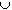#Interactive Real Analysis

Next | Previous | Glossary | Map

## 6.4. Topology and Continuity

### Proposition 6.4.4: Images of Compact and Connected sets

If f is a continuous function on a domain D, then:
1. the image of every compact set is again compact.
2. the image of every connected set is again connected.

### Proof:

1. Note that the image of a closed set is not necessarily closed for continuous functions, and the image of a bounded set is not necessarily bounded. However, the image of a close and bounded set is again closed and bounded (under continuous functions). Despite this, the proof is fairly easy: Recall that a set D is compact if every open cover of D can be reduced to a finite subcover.

Let A be an open cover of the set f(D). Since f is continuous, the collection

• {f-1(U): UA}

is a collection of open sets that cover D. Since D is compact, this collection can be reduced to a finite subcover, say:

• f-1( U1), f-1( U2), ..., f-1( Un),

But then the sets U1, U2, ..., Un cover the set f(D). Hence, every open cover of f(D ) can be reduced to a finite subcover. Therefore, f(D ) is compact.

Note: We have used the fact that the inverse image of open sets is open. That, however, is only true if the original domain D of the function is also open. Can you modify this proof so that it still works for a not necessarily open domain D ?

2. This prove is again simple. The idea is as follows: suppose UD is connected, but f(U) is not connected. Then

• f(U ) = AB with A, B open and disjoint

Since f is continuous, f-1(A) and f-1(B) are both open. They are clearly disjoint, and their union makes up all of U. But then U is not connected, which is a contradiction. Thus, the image of every connected set under a continuous function is connected.

Note: Just as before, this proof is not completely correct. It does reflect the major idea, but some technicalities are missing. Why is this proof technically not correct ? Can you fix it ?

Next | Previous | Glossary | Map